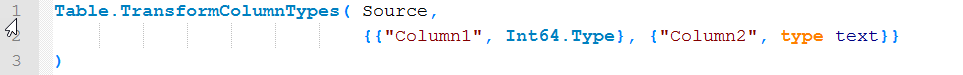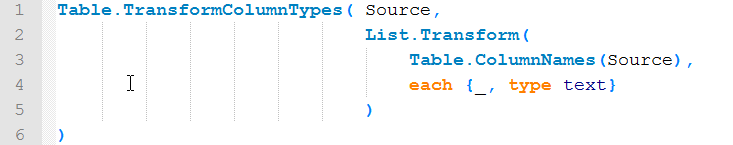# Dynamic & bulk type transformation in Power Query, Power BI and M

This is just a quick code-share-of-the-day of different scenarios for dynamic type transformation of multiple columns at once.

The syntax to transform the format of 2 columns (“Column1” and “Column2”) in a table (“Source”) looks like this:Now if you would like to do one of the following:

### 1. Force all columns of the table to be transformed to one type (text)The second argument of Table.TransformColumnTypes is a list of lists, whose elements contain 2 arguments: The name of the column to transform and the type to be applied.

For this dynamic approach we start with a list of the table’s column names (Table.ColumnNames) and transfer it magically to a list of list, using List.Transform with an expression with curly brackets again like this: each {_, type text}: This operation iterates through every element of the list (List.Transform) and performs the actions that follow the “each” on every element of the list (which is represented by the underscore: _)

### 2. Transform all newly added columns of a table to one specific type

Imagine you have a table with different column types where users can add new columns with random names. You want these columns automatically to be converted to text:Same procedure as the first, just that you need to identify the newly added column names. Therefore you use List.Intersect with the two tables to compare in list-format (curly brackets) as shown in line 3 above.

### 3. Transform all columns whose name are in a list to one specific type

Let’s close with the easiest case, which you’d probably be able to find out by yourself: Say your query returns a dynamic list somewhere with column names who then shall all be converted to a specific type:You can directly reference the List as the first argument of the List.Transform-command.

Enjoy & stay queryious 🙂

### Comments (29) Write a comment

1.Thank you, Imke!
Here’s something you might be interested in. It demonstrates dynamic table creation ( Column Names, Column Types and values ) via parameter table and recursive function. This WIP lacks defensive code and may not account for ALL primitive types but you’ll get the gist. Just plop in editor and go; it seems to restore original formatting. 🙂 Maybe you have some thoughts on this as well ( last few posts ):

https://www.powerpivotpro.com/2016/12/how-many-working-days-has-an-employee-been-off-work/

Many thanks, again.

let
Source = Table.FromRecords( {
[ Root Symbol = “A”],
[ Root Symbol = “AA” ],
[ Root Symbol = “AAC” ],
[ Root Symbol = “AAL” ],
[ Root Symbol = “AAN” ],
[ Root Symbol = “AA” ],
[ Root Symbol = “AAOI” ],
[ Root Symbol = “AAON” ],
[ Root Symbol = “AAP” ],
[ Root Symbol = “AAPL” ]
} ),

SymbolRowCount = Number.IntegerDivide( #”Counted Rows”, 20 ) + (if Number.Mod( #”Counted Rows”, 20 ) > 0 then 1 else 0 ),
PackedSymbolTable_ = Table.FromList({ 1..SymbolRowCount } as list, Splitter.SplitByNothing(), null, null, ExtraValues.Error),
#”Counted Rows” = Table.RowCount( Source ),

let
FinalList_ = List.Range( Source [Root Symbol], ( [Column1] * 20 – 20 ), 20 ),
FinalList = Text.Combine( FinalList_, “+” ) as text
in
FinalList),

YAHOO_Tags = Table.FromRecords( {
[ Tag = “a”, ColumnName = “Ask”, Type = “Currency.Type” ],
[ Tag = “b”, ColumnName = “Bid”, Type = “Currency.Type” ],
//[ Tag = “l1”, ColumnName = “Last”, Type = “Currency.Type” ],//
let
ColumnsTransformed_ = Table.TransformColumnTypes( Table, { NewColNames { Index },
if ( YAHOO_Tags [Type] { Index } = “Currency.Type” ) then Type.ListItem( type { Currency.Type } )
else Type.ListItem( type { text } ) } ),
ColumnsTransformed = if ( Index

let
/* Create an “Error” table with the same schema as a valid quote table */

/* See Chris’s blog on passing parameters to functions within Function.InvokeAfter(): https://blog.crossjoin.co.uk/2015/04/30/using-function-invokeafter-in-power-query/ */
Source_ = Function.InvokeAfter( ()=>
#duration( 0, 0, 0, .5 ) ),
QuoteTable = Csv.Document( Source_, [Delimiter=”,”, Encoding=1252] )
in
QuoteTable

in
FinalTable

2.After learning several ideas from the material you share around the web, today I managed to get 99% of the way there by myself with a solution for case 1 as mentioned above. Upon reviewing your examples here and applying some quick corrections, my code is working. Now I am moving full speed ahead! Thank you for your contributions around the web Imke! Cheers!

•Thanks Parker for this great feedback 🙂
/Imke

3.Can this be adjusted to match the list of column names we want to change a type to with an associated list of types? Out of 30 columns I have a list of 5 column names and associated types (e.g. column 1 –> Int64.Type, column 2 –> type text and so on. I can see in your last example how to iterate through the first list of column names to be changed but I can’t figure out how to match the column name to the corresponding Type in the other list.

•Yes, this can easily be done as well:
If you have your transformations in a table with the column names in the first column and the (real) types in the second column, the formula would be this: Table.TransformColumnTypes(Source, Table.ToRows(Transformations))
But if they are in separate lists, you would have to use List.Zip like so: Table.TransformColumnTypes(Source, List.Zip( { ListWithColumnNames, ListWithTypes } ))

•I have 2 tables with 1 column each. First one with the columnnames, second one with the names of the types. I transfer them to lists like this:

But I get an error:

Expression.Error: We cannot convert the value “type text” to type Type.
Details:
Value=type text
Type=Type

•Your table with types mustn’t contain the textual representation of the type, but the type itself.
Like so for example: #table({“MyTypes”}, {{type text}, {type number}})

•Thank you, that did the trick.

•There is any format to change the Text Type to “Type Type”, i’m trying do what EM are doing with diferent tranformations using one table of transformations

•Sorry, but I don’t understand what you’re trying to achieve. Could you please give more details?
/Imke

•I am also in the same boat as MATEUS – I think the question is: How do you convert a textural list of types into a list of the types themselves?

I am able to get the textural list by doing Table.Schema(Source)[TypeName], but I have no idea how to convert the text list into a list of types to use – specifically for this:

Table.TransformColumnTypes(Source, List.Zip( { ListWithColumnNames, ListWithTypes } ))

•I’m running into the same issue as Mateus and Spizzle. The question is how to dynamically generate real types out of text.

4.Hi Imke!

I’ve implemented this solution and it worked like a charm! Thank you!

I’ve tried using a similar approach to TransformColumns function and I’m not getting the expected result.

I have a table to adjust scale for each field of type integer individually (ScaleTable) and I want to dynamically generate the transformation list to TransformColumns function.
Here’s my code:

let
ScaleTable = #table({“Field”, “Scale”},{{“Price”, 2},{“Rate”, 6}}), //ScaleTable example
ScaleList = Table.ToRows(ScaleTable),

`Source = #table({"Price", "Rate"},{{300, 3000000},{40, 40}}),`

``` TransformList = List.Transform(ScaleList, each {_{0}, each (Value as number) as number => Value / Number.Power(10, _{1})}), ResultTable = Table.TransformColumns(Source, TransformList), //dynamic TransformColumns generate functions instead of its return value ```

```ExpectedTable = Table.TransformColumns(Source, {{"Price", each _ / Number.Power(10, 2)}, {"Rate", each _ / Number.Power(10, 6)}}) ```

in
ExpectedTable

In the above code, I’d like to adjust the Price for 2 decimal places and the Rate for 6 decimal places. However, ResultTable doesn’t show the function return value… Instead, it shows the function itself! It’s as if the function is not invoked in TransformColumns function.

Is there any way to handle with it? Or this approach isn’t possible in PQ?

•That’s completely doable.
Just get rid of one “each” in step “TransformList” like so:
TransformList = List.Transform(ScaleList, each {_{0}, each (Value as number) as number => Value / Number.Power(10, _{1})}),

TransformList = List.Transform(ScaleList, each {_{0}, (Value as number) as number => Value / Number.Power(10, _{1})}),

Cheers, Imke

•Perfect!

Thank you so much, Imke! You and your blog are great!

Cheers!

5.As always, your solutions are beautiful and multipurpose. Thanks

•Thank you 🙂

6.Inspired by this post – and a problem from a colleague stating:

“…I need to convert all numeric values into text using a specific format!”

I ended up with this code – hope others can use it:

= Table.TransformColumns(Source, List.Transform(ListOfColumnNames, each {_, (Value as number) as text => Number.ToText(Value, “n”, “1033”)}))

•Well done & thanks for sharing!

•= Table.TransformColumns(Source, List.Transform(ListOfColumnNames, each {_, (Value as nullable number) as nullable text => Number.ToText(Value, “n”, “1033”)}))

•Thanks Claus!

•Do you know how to dynamically trim each column? I tried test = Table.TransformColumns(Source, List.Transform(Table.ColumnNames(Source), each {_, (Value as text) as text => Text.Clean(Value)}))

7.Danke Schön Imke (and Daniil, I used his code in the comments), this works great. I’m retrieving snapshots from Azure Datalake Storage Gen2 where CSVs are “naked data” with column headers listed in a separate model.json file, with this my code will be accomodate schema changes automatically.

•Great – thanks for letting us know 😉

8.Nice article, thanks !

I had a similar question as Mateus , Spizzle, otravers

I wanted to be able to programatically affect the type of the columns in my table, based on a parameter table where I enter the desired type for each column.

I found inspiration in your article and in this post : https://community.powerbi.com/t5/Desktop/change-column-type-programmatically/td-p/140534

Here is the solution that works for me, if it can help :

// Some data :
let
Source = #table({“Sales”,”Currency”},{{“1000″,”EUR”},{“100″,”EUR”}})
in
Source

// I define the type in a parameter table :
let
Source = #table(type table[ColumnName = text, ColumnType = text],{ {“Sales”, “type number”}, {“Currency”, “type text”} }),
TextToType = Table.TransformColumns(Source,{{“ColumnType”, Expression.Evaluate}})
in
TextToType

// Define the type for each column with the desired type :
let
in
Source

••